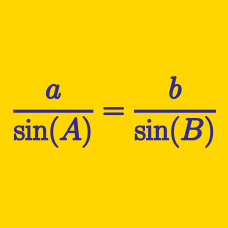Geometry

# Sine Rule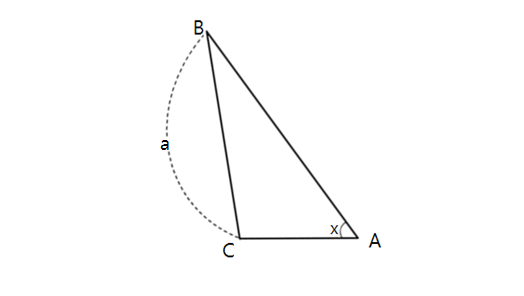In triangle $ABC$ above, $\angle BAC =x= 30^{\circ}, \sin \angle ABC = \frac{1}{4}, \lvert \overline{BC} \rvert =a= 9,$ where $\lvert \overline{BC} \rvert$ is the length of $\overline{BC}.$ What is the value of $\lvert \overline{AC} \rvert^2?$

Triangle $ABC$ has angles $\angle A = 60^{\circ}$ and $\angle B = 45^{\circ}$, and a side length $a = 21 \sqrt{6}$. What is the side length $b$?

Details and assumptions

$a$ and $b$ are the lengths of the sides opposite to the vertices $A$ and $B$, respectively.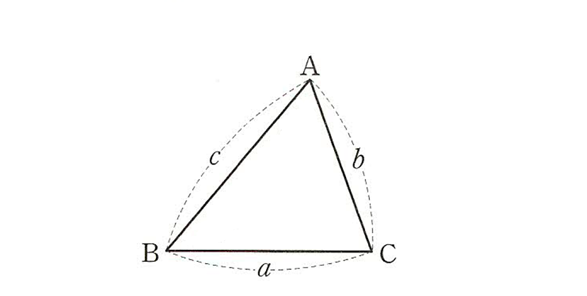In triangle $ABC$ above, we are given the side lengths as follows:$\lvert\overline{BC}\rvert=a, \lvert\overline{CA}\rvert=b, \lvert\overline{AB}\rvert=c.$ If $ab : bc : ca = 2 : 11 : 5,$ what is the value of $\frac{\sin A + \sin B}{\sin C}?$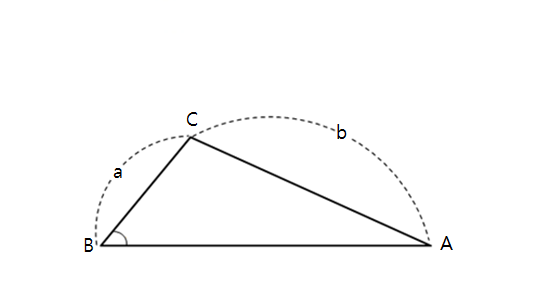In triangle $ABC$ above, $\angle ABC =x= 30^{\circ}$ and $\lvert \overline{BC} \rvert =a= 6, \lvert \overline{CA} \rvert =b= 10.$ What is the value of $\left(\sin \angle BAC\right)^2?$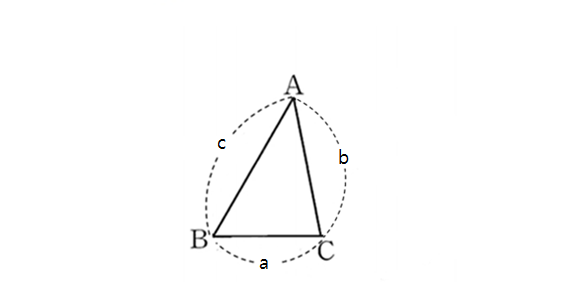In triangle $ABC$ above, we are given the side lengths as follows:$\lvert\overline{BC}\rvert=a, \lvert\overline{CA}\rvert=b, \lvert\overline{AB}\rvert=c.$ If $\sin A : \sin B = 5 : 4$ and $\ a : c = 2 : 5,$ what is $a : b : c?$

Note: The above diagram is not drawn to scale.

×